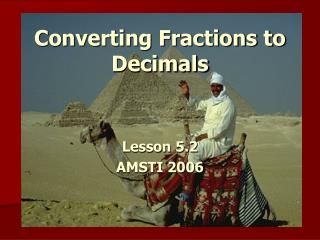DownloadDownload PresentationConverting Fractions to Decimals

# Converting Fractions to Decimals

Télécharger la présentation## Converting Fractions to Decimals

- - - - - - - - - - - - - - - - - - - - - - - - - - - E N D - - - - - - - - - - - - - - - - - - - - - - - - - - -
##### Presentation Transcript

1. Converting Fractions to Decimals Lesson 5.2 AMSTI 2006

2. Discussion • Who can explain what a fraction is? • Who can explain what a decimal is? • Can you find a decimal that is equivalent to ¼? • What method did you use to find this?

3. Complete the following within 1 minute. Compare 5/13 to 4/9 Compare 0.384 to 0.4 Problem of the Day

4. Egyptian Fractions • The Egyptians of 3000 BC had an interesting way to represent fractions.Although they had a notation for 1/2 and 1/3 and 1/4 and so on (these are called reciprocals or unit fractions since they are 1/n for some number n), their notation did not allow them to write 2/5 or 3/4 or 4/7 as we would today. Instead, they were able to write any fraction as a sum of unit fractions where all the unit fractions were different. • A fraction written as a sum of distinct unit fractions is called an Egyptian Fraction.

5. Why use Egyptian Fractions Today? • For two very good reasons: • The first reason is a practical one. Suppose you have 5 sacks of grain to share between 8 people, so each would receive 5/8 of a sack of grain in terms of present-day fractions. How are you going to do it simply, without using a calculator? You could try pouring the 5 sacks of grain into 8 heaps and, by carefully comparing them, perhaps by weighing them against each other, balance them so they are all the same! But is there a better way? We will see that using unit fractions makes this easier. • The second reason is that it is much easier to compare fractions using Egyptian fractions than it is by using our present-day notation for fractions! For instance: Which is bigger: 5/8 or 4/7? • but remember - you are notallowed to use your calculator to answer this! Again unit fractions can make this much simpler. • On this page we see how both of these work in Egyptian fractions.

6. Video Lets watch a video to preview how to convert fractions to decimals, the Egyptian way!

7. Converting Fractions to Decimals • To convert a Fraction to a Decimal manually, follow these steps: • Step 1: Find a number you can multiply by the bottom of the fraction to make it 10, or 100, or 1000, or any 1 followed by 0s. • Step 2: Multiply both top and bottom by that number. • Step 3. Then write down just the top number, putting the decimal place in the correct spot (one space from the right for every zero in the bottom number)

8. Method #1: Converting with Proportions • Example # 1: Express 3/4 as a Decimal • Step 1: We can multiply 4 by 25 to become 100 • Step 2: Multiply top and bottom by 25 3 = 75 4 100

9. Example # 1 continued…. • Step 3: Write down 75 with the decimal place 2 spaces from the right (because 100 has 2 zeros); • Answer = 0.75 • Can you explain what we just did? • Try to express ¼ as a decimal using method # 1.

10. Method #2: Convert by Dividing • Example #1: to write 5/8 as a decimal, we need to calculate 5 ÷ 8: 0.625 8 √ 5.000 So = 0.625 as a decimal. • Try this example: write 4/5 as a decimal using division (method #2).

11. Skittles Activity • Sort your skittles into groups by color. • Find the fraction of each color. • Convert your fractions into a decimals. • Record your data on chart paper. • Compare and discuss with the class.

12. Lets Practice!! • We are going to use this website to fish out some fractions and decimals. • Lets see how well you understand this lesson. • Go to www.iknowthat.com • Select “Math” in the left margin • Select “Fishy Fractions” • Select “Fractions and Decimal Match”

13. If you still don’t understand…